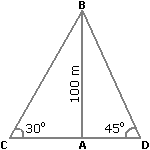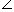# Aptitude - Height and Distance - Discussion

Discussion Forum : Height and Distance - General Questions (Q.No. 1)
1.
Two ships are sailing in the sea on the two sides of a lighthouse. The angle of elevation of the top of the lighthouse is observed from the ships are 30° and 45° respectively. If the lighthouse is 100 m high, the distance between the two ships is:
173 m
200 m
273 m
300 m
Explanation:

Let AB be the lighthouse and C and D be the positions of the ships.Then, AB = 100 m,ACB = 30° andADB = 45°.

 AB = tan 30° = 1AC = AB x 3 = 1003 m. AC 3

 AB = tan 45° = 1AD = AB = 100 m. ADCD = (AC + AD) = (1003 + 100) m = 100(3 + 1) = (100 x 2.73) m = 273 m.

Discussion:
96 comments Page 1 of 10.

Akshay said:   2 months ago
Short tricks
(1)30_60_90
1:√3:2.

So,
a = 1×100.
= 100m.

b = √3×100.
= 173m.

d = 100 × 2
= 200m.

(2) 45_45_90
1:1:√2.

∆dcb
C = 1×100.
= 100m.

B = 1×100,
= 100m.

D = √2×100.
= 141m.

Total
= 273m.
(1)

SAI ESVAR said:   4 months ago
Can anyone explain this problem clearly to understand?
(3)

HECKER said:   7 months ago
Yes, right @Kumar.
(1)

Hemanth said:   7 months ago
Why we use the tan formula? Anyone explain me.
(1)

Kumar said:   2 years ago
For triangle, we use tan mostly. right.

Khaleeq ur rehman said:   2 years ago
According to My observation, when the angle of elevation is 45 degree then base and perpendicular must be equal which is 100 according to AD measurement if another elevation is less then from another which is 30 degree of AC then how is possible that distance/length of AC increase from another distance. Please anyone explain.
(1)

Anudeep said:   2 years ago
Why we are taking √3? Explain please.

Mythili said:   2 years ago
@Yeshi Samdup

How?

CA = 100/tan30,
CA = 173.

(1)

Bhavarth said:   2 years ago
@Kanishka Roy.

The one which is opposite to angle will be height.

And one which is adjacent to angle will be base.

Note that the one which is the base is not the longest side of triangle otherwise it would be the hypotenuse.

Kanishka Roy said:   3 years ago
How do you decide which is the base and which is the height? Explain it, please.